Next: Secant Method Up: Optimization Previous: Method of Conjugate Gradients

## The Newton-Raphson Method

We now devote our attention to a method of category (3) from page. The method differs from the Steepest Descent and Conjugate Gradients method, which both are of category (2), in that the information of the second derivative is used to locate the minimum of the function. This results in faster convergence, but not necessarily less computing time. The computation of the second derivate and the handling of its matrix can be very time-consuming, especially for large systems.

The idea behind the Newton-Raphson method is to approximate the given functionin each iteration by a quadratic function, as given in equation (4.6), and then move to the minimum of this quadratic. The quadratic function for a pointin a suitable neighbourhood of the current point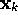is given by a truncated Taylor series:where both the gradient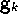and the Hessian matrixare evaluated at. The derivative of (4.15) is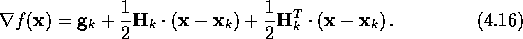The Hessian matrix is always symmetric if the functionis twice continuously differentiable at every point, which is the case here. Hence, (4.16) reduces to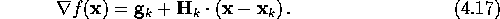If we assume thattakes its minimum at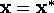, the gradient is zero; i.e.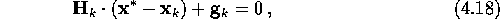which is noting else but a linear system. The Newton-Raphson method uses the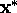as the next current point, resulting in the iterative formulawhere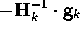is often referred to as the Newton direction.

If the approximation in (4.15) is valid, the method will converge in just a few iterations. For example, if the function to be optimized,, is a n-dimensional quadratic function, it will converge in only one step from any starting point.

Even though the convergence may seem to be fast, judged by the number of iterations, each iteration does include the calculation of the second derivative and handling of the Hessian. The performance of the method is therefore very dependent on certain qualities of the Hessian. One of these qualities is positive definiteness. For the method to converge towards a minimum, the Newton direction needs to be a direction of descent. Thus, we requirewhich, by inserting (4.19), givesThis inequality is satisfied at all points for whichifis positive definite. The furtheris from the solution, the worse the quadratic approximation in (4.15) gets, which can result in the Hessian not being positive definite. The method is then no longer guaranteed to proceed toward a minimum; it may end up at any other critical point, whether it be a saddle point or a maximum point.

There are several ways to make sure that the Hessian is positive definite, e.g. by adding a large enough, whereis the unit matrix andis a positive scalar, or by diagonalizing the matrix by the use of eigenvalues. Descriptions of some of these methods can be found in textbooks like GMW,DS.

The size of the Hessian can also be crucial to the effectiveness of the Newton-Raphson method. For systems with a large number of dimensions, i.e. that the functionhas a large number of variables, both the computation of the matrix, and calculations that includes it, will be very time-consuming. This can be mended by either just using the diagonal terms in the Hessian; i.e. ignoring the cross terms, or just not recalculate the Hessian at each iteration (can be done due to slow variation of the second derivative).

Another serious disadvantage of the Newton-Raphson method is that it is not necessarily globally convergent, meaning that it may not converge from any starting point. If it does converge, it is not unusual that it expend significant computational efforts in getting close enough to the solution where the approximation in (4.15) becomes valid, and the convergence is fast. An aid to this problem is to adjust the stepsize in the Newton direction, assuring the function value to decrease. Equation (4.19) is then as follows: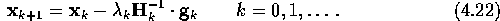There are several methods for doing this, for example linear searches and fixed stepsize mentioned in Subsection 4.3.1, but it is recommended to use a backtracking scheme [e.g. by][]Press, where the full Newton step,, is tried first. If this step fail to satisfy the criterion for the decrease of the function, one backtracks in a systematic way along the Newton direction. The advantage of doing this is that one will be able to enjoy the fast convergence of the Newton-Raphson method close to the solution. For details on the backtracking scheme, see for example [page 377]Press; [Section 6.3]DS.

In spite of these mentioned problems, the Newton-Raphson method enjoys a certain amount of popularity because of its fast convergence in a sufficiently small neighbourhood of the stationary value. The convergence is quadratic, which, loosely speaking, means that the number of significant digits double after each iteration. In comparison, the convergence of Steepest Descent method is at best linear and for the Conjugate Gradients method it is superlinear. The resultant algorithm for the Newton-Raphson method using backtracking is given in Algorithm 4.3.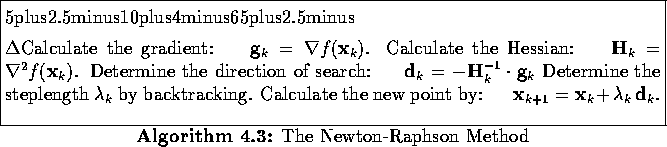As shown in Subsection 4.3.1, the method of Steepest Descent start out having a rather rapid convergence, while Newton-Raphson has just the opposite quality; starts out slowly and ends with a very rapid convergence. It is too obvious to be ignored that these two methods can be combined, resulting in a very efficient method. One starts out with the Steepest Descent method, and switch to the Newton-Raphson method when the progress by the former gets slow, and enjoys the quadratic convergence of the latter. An example of this type of combination is discussed next (and in Section 5.3: the Hybrid method).Next: Secant Method Up: Optimization Previous: Method of Conjugate Gradients

Trond Hjorteland
Mon Jul 5 02:59:28 MET DST 1999